# Electronics and Communication Engineering - Exam Questions Papers

26.

All the four of the 2 x 2 matrix p =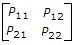are non zero, and one of its eigen values is zero. Which of the following statements is true?

 A. P11P22 - P12P21 = 1 B. P11P22 - P12P21 = - 1 C. P11P22 - P12P21 = 0 D. P11P22 + P12P21 = 1

Explanation:

Use [λI - P] = 0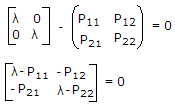⇒(λ - P11)(λ - P22) - P21P12 = 0

Putting λ = 0, we get P11P22 - P21P12 = 0.

27.

The value of the integral of the function g(x, y) = 4x3 + 10y4 along the straight line segment from the point (0, 0) to the point (1, 2) in the x-y plane is

 A. 33 B. 35 C. 40 D. 56

Explanation: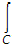(4x3 + 10y4)

y = 2x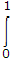[4x3 + 10(2x)4]dx = 33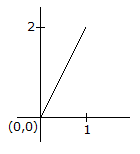28.

For the gear train,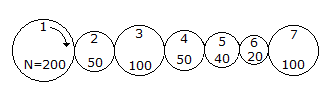If θ1 = 2 rad. clockwise and the torque of gear 1 is 10 lb-ft, what is the displacement and torque of gear 7.

 A.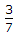rad CW, 7.5 lb-ft B. 4 rad CW, 5 lb-ft C.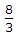rad CW, 7.5 lb-ft D.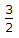rad CW, 7.5 lb-ft

Explanation:

Number of teeths on any gear is proportional to its radius,

i.e.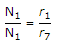and also displacement on all gear must be same

=> r1θ1 = r7θ7

=>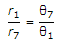=>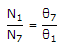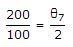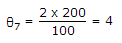rad CW

Now, work done by one gear is equal to other,

T1θ1 = T7θ7

=>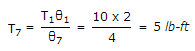.

29.

The following system which is static is __________ .

 A.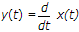B.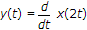C. y(t) = x(n - 1) D. y(t) = ex(t)

Explanation:

The system represented by expression ex(t) is static (memoryless) ∵ output at time = 't' dependent only.

In case of derivative, if we take the laplace transform we have to consider initial conditions.

Hence memory is required.

30.

The minimal function that can detect a "divisible by 3" 8421 BCD code digit (representation D8D4D2D1) is given by

 A. D8D1 + D4D2 + D8D2D1 B. D8D1 + D4D2 D1 + D4D2D1 + D8D4D2D1 C. D4D1 + D4D2 + D8D4 D2D1 D. D4D2D1 + D4D2D1 + D8D4D2D1

Explanation:

Now the problem can be solved using k-map method.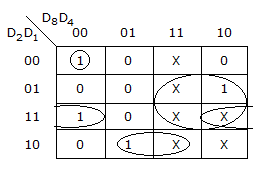The function can be expressed as Σm(0, 3, 6, 9) + d(10, 11, 12, 13, 14, 15)

K-map is

The function is

D8D4D2D1 + D4D2D1 + D4D2D1 + D8D1 .